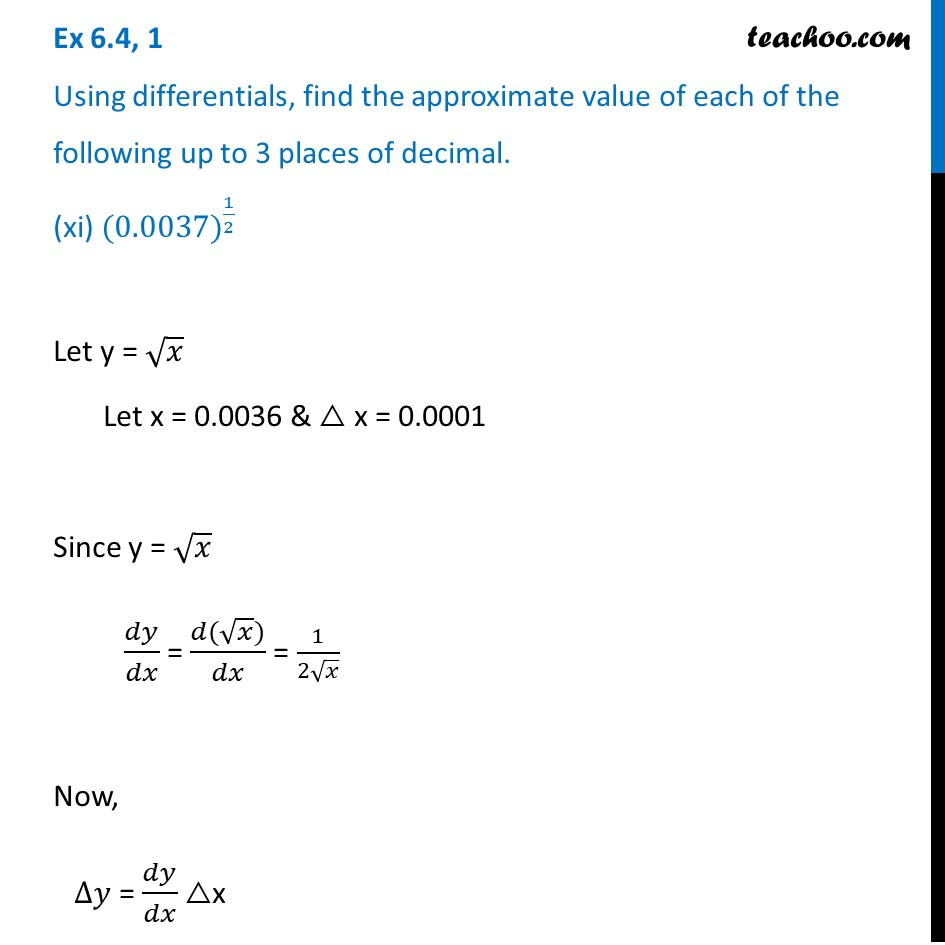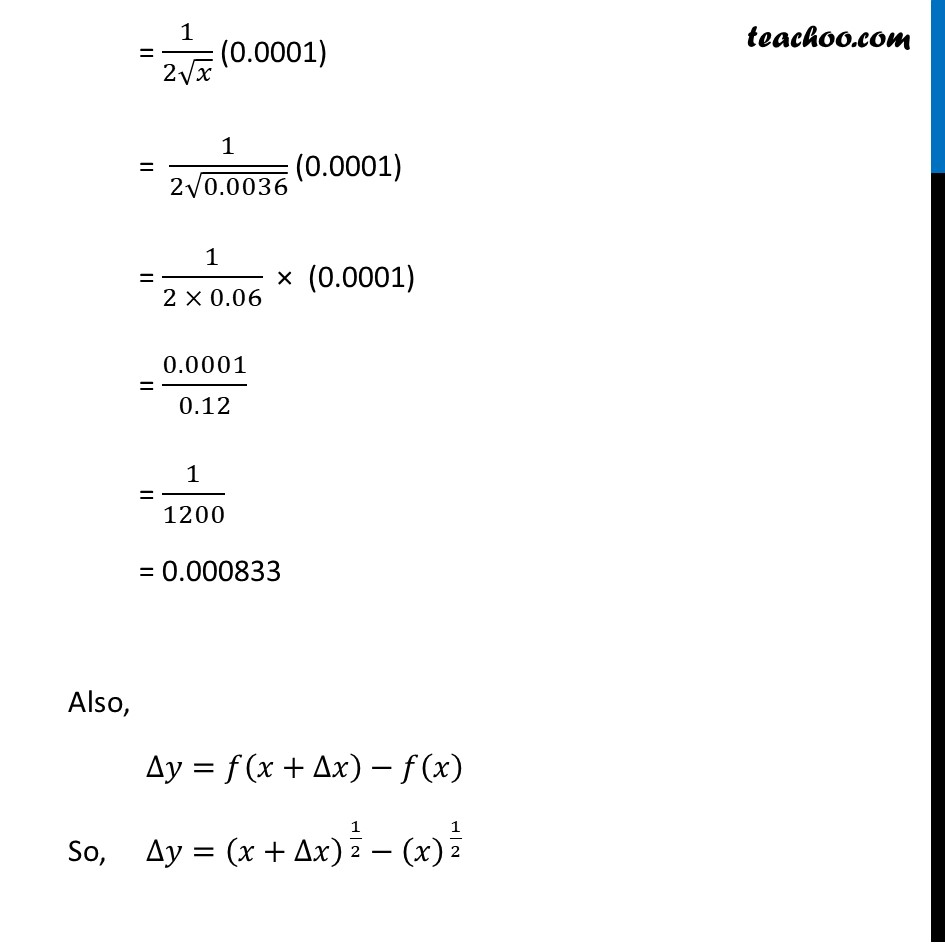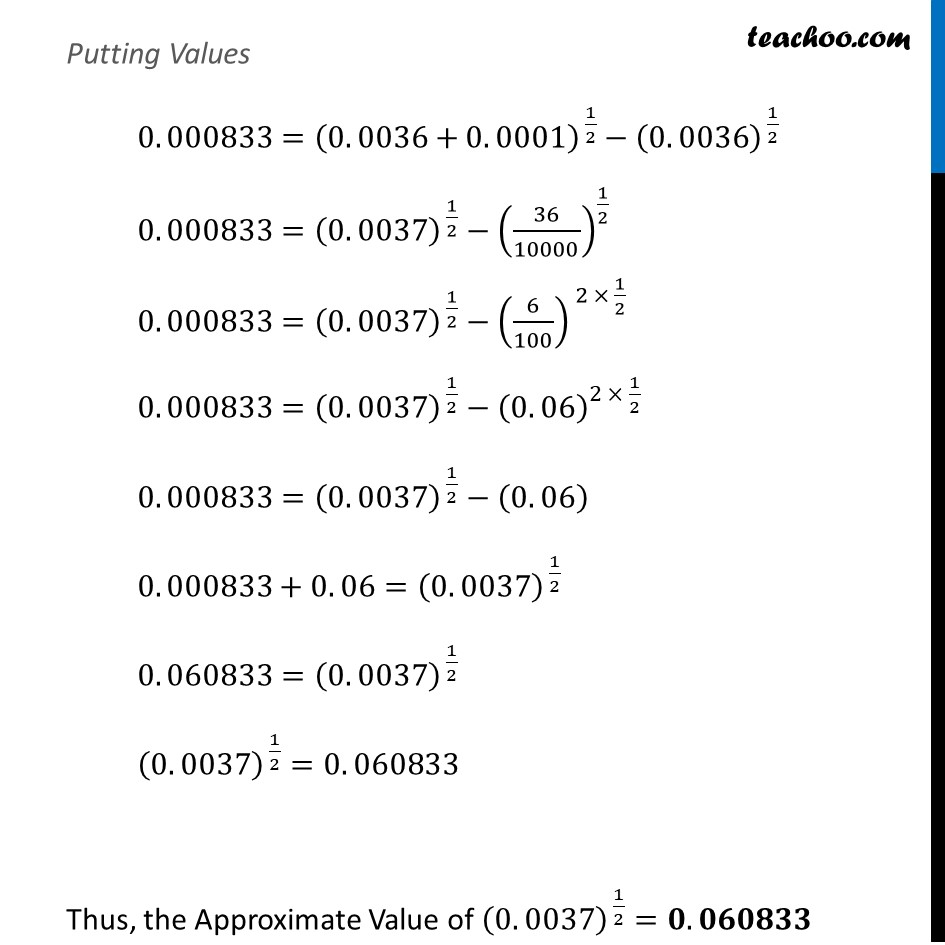Approximations (using Differentiation)

Chapter 6 Class 12 Application of Derivatives
Serial order wiseLearn in your speed, with individual attention - Teachoo Maths 1-on-1 Class

### Transcript

Question 1 Using differentials, find the approximate value of each of the following up to 3 places of decimal. (xi) 〖(0.0037)〗^(1/2)Let y = √𝑥 Let x = 0.0036 & △ x = 0.0001 Since y = √𝑥 𝑑𝑦/𝑑𝑥 = (𝑑(√𝑥))/𝑑𝑥 = 1/(2√𝑥) Now, ∆𝑦 = 𝑑𝑦/𝑑𝑥 △x = 1/(2√𝑥) (0.0001) = 1/(2√0.0036) (0.0001) = 1/(2 × 0.06) × (0.0001) = 0.0001/0.12 = 1/1200 = 0.000833 Also, ∆𝑦=𝑓(𝑥+∆𝑥)−𝑓(𝑥) So, ∆𝑦=(𝑥+∆𝑥)^( 1/2)−(𝑥)^( 1/2) Putting Values 0. 000833=(0. 0036+0. 0001)^( 1/2)−(0. 0036)^( 1/2) 0. 000833=(0. 0037)^( 1/2)−(36/10000)^(1/2) 0. 000833=(0. 0037)^( 1/2)−(6/100)^( 2 × 1/2) 0. 000833=(0. 0037)^( 1/2)−(0. 06)^(2 × 1/2 ) 0. 000833=(0. 0037)^( 1/2)−(0. 06) 0. 000833+0. 06=(0. 0037)^( 1/2) 0. 060833=(0. 0037)^( 1/2) (0. 0037)^( 1/2)=0. 060833 Thus, the Approximate Value of (0. 0037)^( 1/2)=𝟎. 𝟎𝟔𝟎𝟖𝟑𝟑